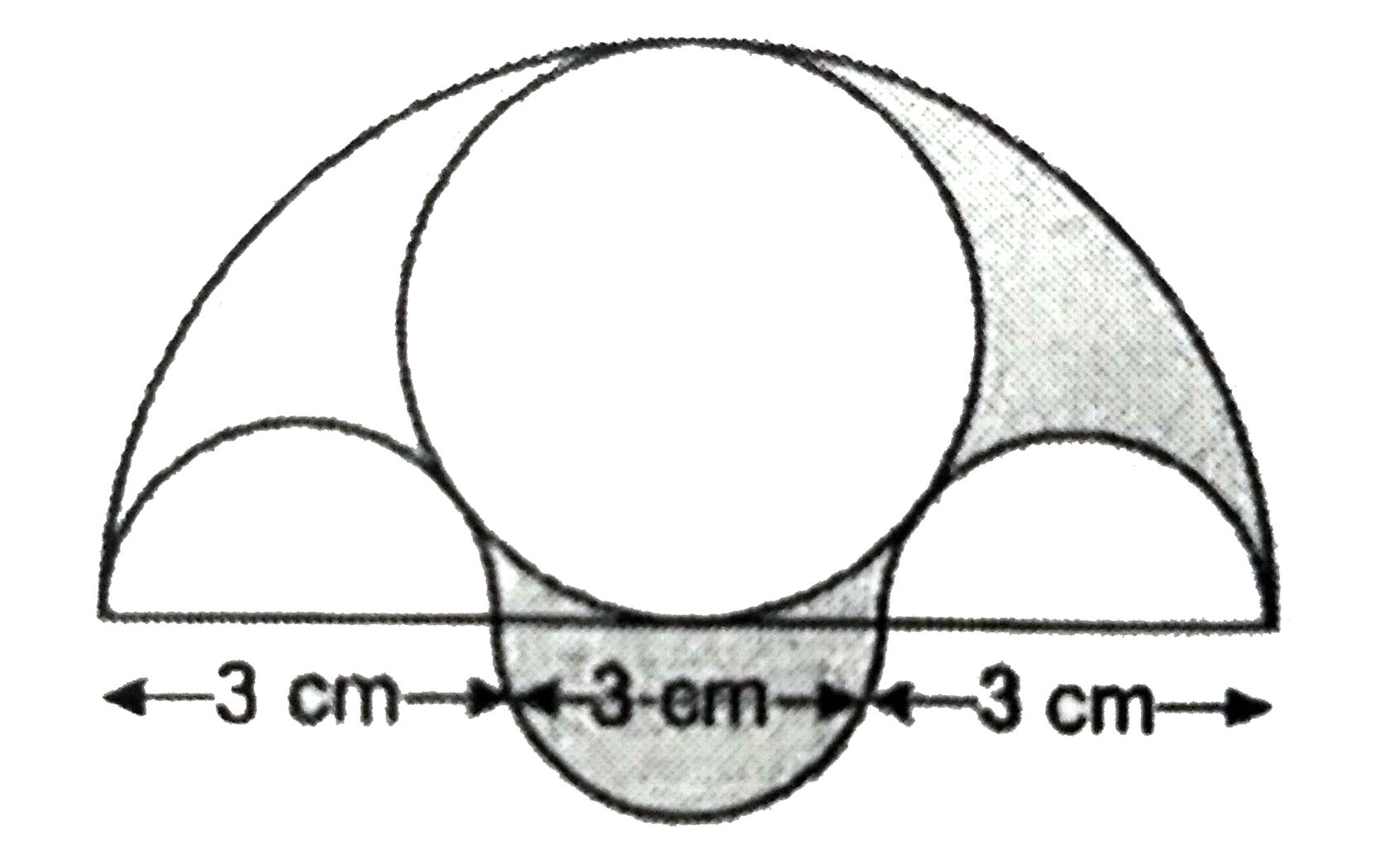# Three semicircles each of diameter 3 cm, a circle of diameter 4.5 cm and a semicircle of radius 4.5 cm are drawn in the adjoining figure. Find the area of the shaded region.Khareedo DN Pro and dekho sari videos bina kisi ad ki rukaavat ke!

Step by step solution by experts to help you in doubt clearance & scoring excellent marks in exams.

Transcript

question is three semicircles each of diameter 3 cm a circle of diameter 4.7 m and a semicircle of radius 5 cm find the area of shaded portion is we have to find the area of the shaded portion here it is a circuit according to the question is this semicircle is remaining parties 3333 semicircles each in semicircle ABC has diameter 3 circle of diameter is diameter is 4.5 CM now I will
its diameter is 3 cm radius of a circle is 1.5 cm is a semicircle b b has also produced in it is because it's given 4.5 cm It means 4.5.2 I get to that radius of a circle of radius 4.5 which is that this
School circle distance and I can say is again its radius is 4.5 and this is all the data from the first area of big circle pictures India Circle now are is 4.5 it means I can write bye-bye to INR to 4.5 converting I have to add this one area of Semi circle
which is it is it is also means value of a beam is 1.5 X 21.5 it is 1.5 square and area of semicircle CCNA area will be exactly La CD Circle to 2.25 find the area of the
semi circle plus this credit card it can be written area we have to add the area of a + b and will exclude a and c and now we will put all the E + 1 upon 2 + b is 2.5 whole square + 3 + 1.5 whole it 1.25 taking common 541
because it is the area of circle D it is a closet and I love you will torque calculation to get 1.254 254.254 51 again 2.2.52 5.1.2 buy into adding 22.50
1.25 in 2.5.0 625 solving between after cancel Appam to 11.25 after cancer light but point 255 it is -5.25 now I will be taking com 11.25 it means if we put value is 20 to solve it will be 8.93
12.5 CM of the shaded Pole is 12.3 75

Related Videos